# Implicit function (in algebraic geometry)

A function given by an algebraic equation. Let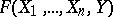be a polynomial inand(with complex coefficients, say). Then the varietyof zeros of this polynomial can be regarded as the graph of a correspondence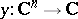. This correspondence, allowing for a certain impreciseness, is also called the function given implicitly by the equation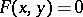. Generally speaking,is many-valued and not defined everywhere and so is not a function in the usual sense. There are two ways of turning this correspondence into a function. The first, which goes back to B. Riemann, consists in assuming that the domain of definition of the implicit functionis notbut the variety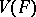, which is a finite-sheeted covering of. This device leads to the highly important concept of a Riemann surface. In this approach the notion of an implicit function interlinks with that of an algebraic function.

The other approach consists in representinglocally as the graph of a single-valued function. Various implicit-function theorems assert that there are open setsandfor whichis the graph of a smooth function (in one sense or another)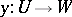(see Implicit function). However, the open setsandare, as a rule, not open in the Zariski topology and have no meaning in algebraic geometry. Therefore, one modifies this method in the following manner. A formal germ (or branch) at a point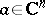of the implicit function given by the equation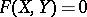is defined as a formal power series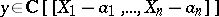such that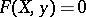. Quite generally, a power seriessatisfying a polynomial equationis said to be algebraic. An algebraic power series converges in a certain neighbourhood of.

Letbe a local Noetherian ring with maximal ideal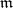. An elementof the completion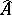ofis said to be algebraic overiffor some polynomial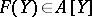. The set of elements ofthat are algebraic overforms a ring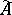. The following version of the implicit-function theorem shows that there are sufficiently many algebraic functions. Letbe a collection ofpolynomials from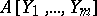and letbe elements of the residue class field(the bar above a letter means reduction) such that:

1)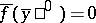;

2).

Then there exist elements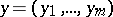algebraic oversuch that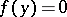and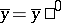. In other words,is a Hensel ring.

Another result of this type is Artin's approximation theorem (see ). Letbe a local ring that is the localization of an algebra of finite type over a field. Next, let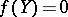be a system of polynomial equations with coefficients in(or in) and letbe a vector with coefficients insuch that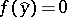. Then there is a vectorwith components in, arbitrarily close to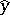and such that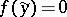. There is also a version  of this theorem for systems of analytic equations.